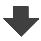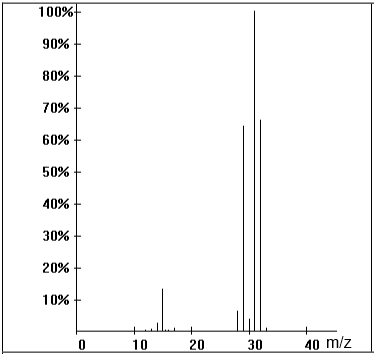Search:

# Mass spectroscopy

## Exercise 2

Identify the base peak and the molecular peak of the following mass spectrum. What is the molar mass of the substance?$\frac{m}{z}$ $=$ $31$ $\Rightarrow$: base peak $\frac{m}{z}$ $=$ $32$ $\Rightarrow$ molecular peak $M$ $=$ $32 \frac{g}{mol}$

What causes the peak $\frac{m}{z}$ $=$ $32$ ?

This peak is probably due to the isotope $^{13}C$ present in the substance.

What causes the peak $\frac{m}{z}$ $=$ $15$ ?

This peak is probably due to the ion $CH_3^+$

Do you see a very specific peak?

The peak $\frac{m}{z}=31$ is due to the ion $CH_2OH^+$

What substance is this?

Methanol $CH_3OH$